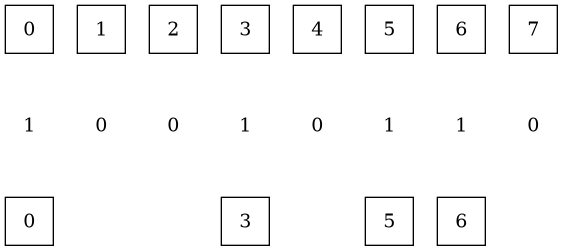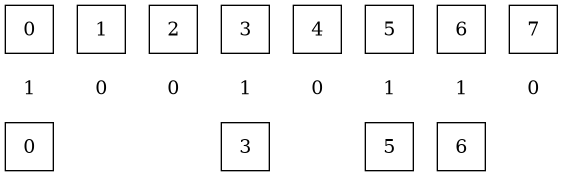# Problem

Is there a rank in-between `source` and `sink`?

It’d be nice to take nominal values (e.g., `rank=1`, `rank=2`,
`rank=3`).

# MWE

## Have

``````digraph subset {
// rankdir="LR"
node [label="", shape="square"]
edge [style="invis"]

subgraph fullset {
rank="source"

fs0 [label="0"]
fs1 [label="1"]
fs2 [label="2"]
fs3 [label="3"]
fs4 [label="4"]
fs5 [label="5"]
fs6 [label="6"]
fs7 [label="7"]

fs0 -> fs1 -> fs2 -> fs3 -> fs4 -> fs5 -> fs6 -> fs7
}

subgraph rn {
rank="sink"
node [shape="none"]

r0 [label="1"]
r1 [label="0"]
r2 [label="0"]
r3 [label="1"]
r4 [label="0"]
r5 [label="1"]
r6 [label="1"]
r7 [label="0"]

r0 -> r1 -> r2 -> r3 -> r4 -> r5 -> r6 -> r7
}

subgraph subset {
rank="min"

s0 [label="0"]
s3 [label="3"]
s5 [label="5"]
s6 [label="6"]

node [style="invis"]
s1
s2
s4
s7

s0 -> s1 -> s2 -> s3 -> s4 -> s5 -> s6 -> s7
}
}
``````

## Want

`fullset` up top, `rn` in middle, `subset` on bottom (in order that they
appear).

Produced the opposite by removing ranks and specifying `rankdir`:

For the general case, no (see Allow user to provide levels, or levels plus ordering (no ranking or crossing minimization), and let dot finish (#2023) · Issues · graphviz / graphviz · GitLab).
But for a 3rd / middle rank, yes (rank | Graphviz):
rank=source // exclusive
rank=same // all the rest
rank=sink // exclusive

`same` actually does the trick!

Glad to see the idea of levels is being considered.

The problem remaining is that `node [shape="none"]` changes the size (as you pointed out in my other post) and `node [style="invis"]` also makes the label invisible.

I set `square` and `none` to `0.5`. I believe that’s what `square` is anyway, but hard-coded it for my sanity.

Ah, now it’s pretty spaced out though:I want to decrease the vertical spacing. I tried `ranksep=0.5` at the top of the digraph but to no avail.

That’s cause `0.5` is the default.

`0.1` did it.

Here is the final working solution:

``````digraph subset {
ranksep=0.1
node [shape="square", width=0.5, label=""]
node [shape="square", label=""]
edge [style="invis"]

subgraph fullset {
rank="source"

fs0 [label="0"]
fs1 [label="1"]
fs2 [label="2"]
fs3 [label="3"]
fs4 [label="4"]
fs5 [label="5"]
fs6 [label="6"]
fs7 [label="7"]

fs0 -> fs1 -> fs2 -> fs3 -> fs4 -> fs5 -> fs6 -> fs7
}

subgraph rn {
rank="same"
node [shape="none", width=0.5]

r0 [label="1"]
r1 [label="0"]
r2 [label="0"]
r3 [label="1"]
r4 [label="0"]
r5 [label="1"]
r6 [label="1"]
r7 [label="0"]

r0 -> r1 -> r2 -> r3 -> r4 -> r5 -> r6 -> r7
}

subgraph subset {
rank="sink"

s0 [label="0"]
s3 [label="3"]
s5 [label="5"]
s6 [label="6"]

node [style="invis"]
s1
s2
s4
s7

s0 -> s1 -> s2 -> s3 -> s4 -> s5 -> s6 -> s7
}
}
``````Marking this as solution for completeness, but wouldn’t have arrived at this solution without @steveroush’s help.